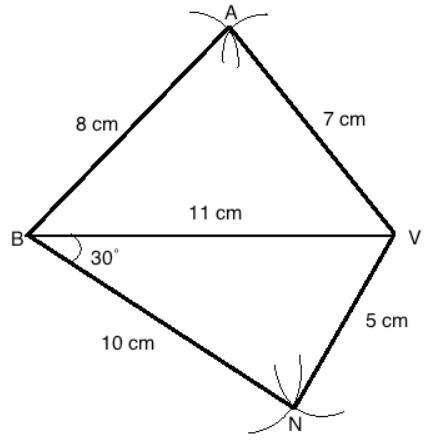QUESTION

# Construct a quadrilateral ABNV, where AV = 7cm, BA = 8cm, BN = 10cm, NV = 5cm, and BV = 11 cm. The relation between diagonals AN and BV isA. AN > BVB. AN < BVC. AN = BVD. None of the above

Hint: This is a problem of construction. Go step by step and label correctly to avoid confusion. The final figure is given below.

The steps to construct are as follows-
1. Make a line 11 cm in length and mark as BV.
2. Make an arc from B 8 cm in length and another arc from V 7 cm in length.
3. The point where they meet is A.
4. Join BA and VA
5. Similarly, make an arc from B 8 cm in length and another arc from V 7 cm in length.
6. The point where they meet is N.
7. Join BN and VN.

After completing the figure, measure the length of AN. It is found that AN > BV.

Hence, the correct option is A. AN > BV.Note: We can take any line as the base. In this case the diagonal was taken as the base for simplicity. For any other base, with properly labelling and measurement, the final result would come out to be the same.